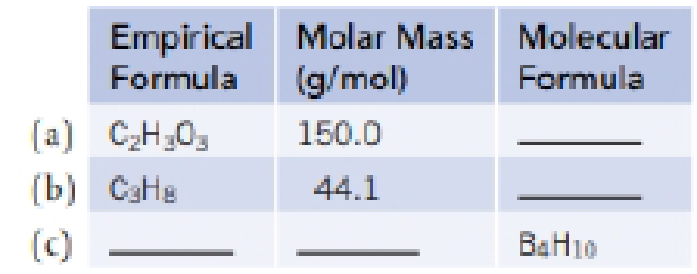# Complete the following table:### Chemistry & Chemical Reactivity

9th Edition
John C. Kotz + 3 others
Publisher: Cengage Learning
ISBN: 9781133949640

#### Solutions

Chapter
Section### Chemistry & Chemical Reactivity

9th Edition
John C. Kotz + 3 others
Publisher: Cengage Learning
ISBN: 9781133949640
Chapter 2, Problem 86PS
Textbook Problem
3 views

## Complete the following table:(a)

Interpretation Introduction

Interpretation:

The table of empirical formula, molar mass and molecular formula are needed to be completed for the given molecule.

Concept introduction:

• Empirical formula of a compound represents the smallest whole number relative ratio of elements in that compound.
• Equation for finding Molecular formula from the empirical formula,

Molecular formula=MolarmassEmpiricalformula mass × Empirical formula

• Equation for number moles from mass and molar mass,

Numberofmoles=MassingramsMolarmass

### Explanation of Solution

For the given empirical formula C2H3O3,

The empirical formula mass of compound is 75.04g

The molar mass of compound is 150g.

Equation for finding Molecular formula from the empirical formula,

Molecular formula=MolarmassEmpiricalformula mass &

(b)

Interpretation Introduction

Interpretation:

The table of empirical formula, molar mass and molecular formula are needed to be completed for the given molecule.

Concept introduction:

• Empirical formula of a compound represents the smallest whole number relative ratio of elements in that compound.
• Equation for finding Molecular formula from the empirical formula,

Molecular formula=MolarmassEmpiricalformula mass × Empirical formula

• Equation for number moles from mass and molar mass,

Numberofmoles=MassingramsMolarmass

(c)

Interpretation Introduction

Interpretation:

The table of empirical formula, molar mass and molecular formula are needed to be completed for the given molecule.

Concept introduction:

• Empirical formula of a compound represents the smallest whole number relative ratio of elements in that compound.
• Equation for finding Molecular formula from the empirical formula,

Molecular formula=MolarmassEmpiricalformula mass × Empirical formula

• Equation for number moles from mass and molar mass,

Numberofmoles=MassingramsMolarmass

### Still sussing out bartleby?

Check out a sample textbook solution.

See a sample solution

#### The Solution to Your Study Problems

Bartleby provides explanations to thousands of textbook problems written by our experts, many with advanced degrees!

Get Started

Find more solutions based on key concepts
All of the following statements concerning beer are correct except beer is poor in minerals beer is poor in vit...

Nutrition: Concepts and Controversies - Standalone book (MindTap Course List)

6.45 Define the term shielding.

Chemistry for Engineering Students

What evidence can you give that granulation is caused by convection?

Horizons: Exploring the Universe (MindTap Course List)

In what way has biotechnology had an impact on agriculture in the United States?

Human Heredity: Principles and Issues (MindTap Course List)

2. Why is carbon unique?

Chemistry In Focus

What physical property is characteristic of optically active molecules?

Chemistry for Today: General, Organic, and Biochemistry

How did echo sounding and an understanding of radiometric dating influence the debate?

Oceanography: An Invitation To Marine Science, Loose-leaf Versin

Suppose you are standing directly behind someone who steps back and accidentally stomps on your foot with the h...

Physics for Scientists and Engineers, Technology Update (No access codes included)